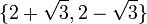# Element structure of special linear group:SL(2,5)

View element structure of particular groups | View other specific information about special linear group:SL(2,5)

This article gives detailed information about the element structure of special linear group:SL(2,5), which is a group of order 120.

## Conjugacy class structure

### Conjugacy classes

Nature of conjugacy class Eigenvalue pairs of all conjugacy classes Characteristic polynomials of all conjugacy classes Minimal polynomials of all conjugacy classes Size of conjugacy class (generic odd$q$) Size of conjugacy class ($q = 5$) Number of such conjugacy classes (generic odd$q$) Number of such conjugacy classes ($q = 5$) Total number of elements (generic odd$q$) Total number of elements ($q = 5$) Representative matrices (one per conjugacy class)
Scalar$\{ 1, 1 \}$ or$\{ -1,-1\}$$x^2 - 2x + 1$ or$x^2 + 2x + 1$$x - 1$ or$x + 1$ 1 1 2 2 2 2$\begin{pmatrix} 1 & 0 \\ 0 & 1 \\\end{pmatrix}$ and$\begin{pmatrix} -1 & 0 \\ 0 & -1\\\end{pmatrix}$
Not diagonal, Jordan block of size two$\{ 1, 1 \}$ or$\{ -1,-1\}$$x^2 - 2x + 1$ or$x^2 + 2x + 1$$x^2 - 2x + 1$ or$x^2 + 2x + 1$$(q^2 - 1)/2$ 12 4 4$2(q^2 - 1)$ 48 [SHOW MORE]
Diagonalizable over field:F25, not over field:F5. Must necessarily have no repeated eigenvalues.$\{ 2 + \sqrt{3}, 2 - \sqrt{3} \}$ and$\{ -2 + \sqrt{3}, -2 - \sqrt{3} \}$, where$\sqrt{3}$ is interpreted a an element of field:F25 that squares to 3$x^2 - x + 1$,$x^2 + x + 1$$x^2 - x + 1$,$x^2 + x + 1$$q(q - 1)$ 20$(q - 1)/2$ 2$q(q - 1)^2/2$ 40$\begin{pmatrix}0 & -1\\ 1 & 1\\\end{pmatrix}$,$\begin{pmatrix}0 & -1\\ 1 & -1\\\end{pmatrix}$
Diagonalizable over field:F5 with distinct diagonal entries$\{ 2,3 \}$$x^2 + 1$$x^2 + 1$$q(q+1)$ 30$(q - 3)/2$ 1$q(q+1)(q-3)/2$ 30$\begin{pmatrix} 2 & 0 \\ 0 & 3 \\\end{pmatrix}$
Total NA NA NA NA NA$q + 4$ 9$q^3 - q$ 120 NA